Multivariable Calculus : Double Integration

Example Questions

Example Question #1 : Double Integration

Calculate the following Integral.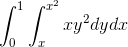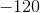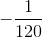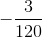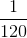Explanation: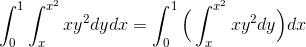Lets deal with the inner integral first.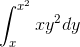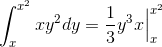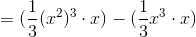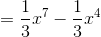Now we evaluate this expression in the outer integral.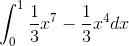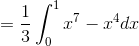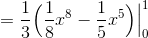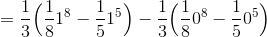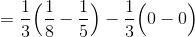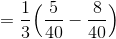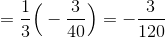Example Question #2 : Double Integration

Calculate the following Integral.Explanation:Lets deal with the inner integral first.Now we evaluate this expression in the outer integral.